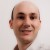# Sigma Versus Sigma – Which is Which?

Six Sigma – iSixSigma Forums Old Forums General Sigma Versus Sigma – Which is Which?

Viewing 6 posts - 1 through 6 (of 6 total)
• Author
Posts
• #29748

Hi!
I am having difficulty understanding something that is probably straight forward. Sometimes I hear people talking about the process sigma and other times I hear them talking about a mathematical sigma. What’s the difference and how can I (as a layperson) quickly understand which one they’re talking about? I’m frustrated! I know of only one sigma — the greek symbol that I learned in high school math. Thanks for your help with a novice. :)
Tina

0
#76730Marc Richardson
Participant

Tina,
Sigma is a measure of dispersion or spread of a population, in other words, its variability. A Population is a general term and can be applied to groups of people (such as age, weight, height, income, etc.), agriculture (crop yield, maturation date, etc.) or the output of a manufacturing process. Process sigma is a measure of the variability of a process, either measured directly (Pressure, speed, voltage, etc) or by the process output (weight, concentration, length, etc). The term sigma is synonymous with standard deviation. It is calculated from all the measurements for every member of the population, as opposed to the estimate of sigma (also known as sigma hat; the greek letter sigma with a caret [^] over it) or the sample standard deviation which is calculated from a sample drawn from the population.
Marc Richardson
Sr.Q.A. Eng.

0
#76732

Thanks, Mark. I really appreciate your help. I thought that was the case, but wasn’t sure.
So if I were to summarize,
Sigma (capital “S”): Process Sigma measures variability or output of a process.
sigma (lowercase “s”, known as the greek letter sigma with a caret [^] over it):  The standard deviation calculated from every member of the population.
sigma estimate (also lowercase “s”, known as the greek letter sigma with a caret [^] over it):  The standard deviation calculated from a sample of the population.
Is that a fair way to describe the differences? Thanks for your help.
Tina

0
#76737Gabriel
Participant

Tina:
Short answer: That was not a fair way do describe the differences.
Sigma (greek leter with no hat) = population’s standard deviation (calcualted from every member of the population). If the population is a process output or a process parameter, the you have a “process sigma”. Hence, ther is no difference between “population sigma” and “process sigma”.
Sigma hat (the greek letter with a ^) = estimation of sigma. If you estimate that sigma is 10 you can not say “sigma”=10, but it is Ok to say “sigma hat”=10.
S (also s) = sample’s standard deviation (calculated only form some members of the population). S is only one from many ways of estimating sigma.
Long asnwer:
Sigma, the greek letter with no hat, is the standard deviation calculated from every member of the population. If your population is “the weight of american men” then sigma is the “weight of american men’s sigma”. If your population is the “volume of coca-cola in the bottles after the filling process” then you have the “volume of coca-cola in the bottles after the filling process sigma”. If it is clear that we are talkng about the volume after the filling proces, we can save words and just say the it is the filling process sigma. Process sigma is just a population sigma where the population is a process parameter or a process output.
Sigma hat, the greek leter with a [^] on it, is an etimation of sigma based on data from a sample. It is used because you ussualy do not measure every members of the population. It is ussually very hard and expensive, and sometimes impossible. If the population is the result of throwing a dice, you have infinite members because you can throw the dice inminite times. So it is impossible to measure all the members. But you can measure let’s say 1000 members and get an estimation for the population. For example, if you estimate the standard deviatin of the population from data you got from a smple, this is sigma hat. One of the estimators used for the population’s standard deviation is the sample’s standard deviation, which is called S. There are other ways to estimate the population’s standard deviation from sample data, not only S (for example, you have Rbar/d2).

0
#76766

0
#76768Arun Nair
Participant

Hi Tina,
Definition #1: Sigma is your standard deviation – the sigma you encountered in your high school math, and which you are probably familiar with.
Definition #2: When people talk about their process capability being 2 sigma or 3 sigma, what it implies is that they can fit 2 or 3 standard deviations of their process between the specification limits ( upper specifiation limit and lower specification limits ). In other words the higher the process capability, the more the number of standard deviations you could fit between the specification limits; the tighter your process spread; in other words a better process.
Thus the mathematical sigma and the process sigma have the same basis.
regards,
arun

0
Viewing 6 posts - 1 through 6 (of 6 total)

The forum ‘General’ is closed to new topics and replies.